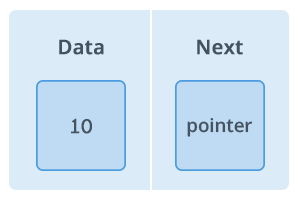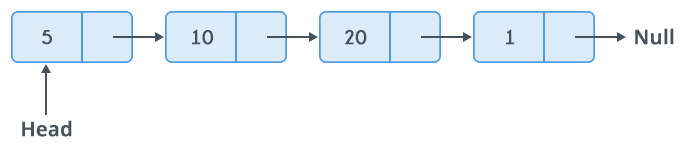Data Structures
Topics:
• Arrays
• Stacks
• Queues
• Hash Tables
• Trees
• Disjoint Data Structures

A linked list is a way to store a collection of elements. Like an array these can be character or integers. Each element in a linked list is stored in the form of a node.

Node:A node is a collection of two sub-elements or parts. A data part that stores the element and a next part that stores the link to the next node.A linked list is formed when many such nodes are linked together to form a chain. Each node points to the next node present in the order. The first node is always used as a reference to traverse the list and is called HEAD. The last node points to NULL.

In C language, a linked list can be implemented using structure and pointers .

struct LinkedList{
int data;
};


The above definition is used to create every node in the list. The data field stores the element and the next is a pointer to store the address of the next node.

Noticed something unusual with next?

In place of a data type, struct LinkedList is written before next. That's because its a self-referencing pointer. It means a pointer that points to whatever it is a part of. Here next is a part of a node and it will point to the next node.

Creating a Node:

Let's define a data type of struct LinkedListto make code cleaner.

typedef struct LinkedList *node; //Define node as pointer of data type struct LinkedList

node createNode(){
node temp; // declare a node
temp = (node)malloc(sizeof(struct LinkedList)); // allocate memory using malloc()
temp->next = NULL;// make next point to NULL
return temp;//return the new node
}


typedef is used to define a data type in C.

malloc() is used to dynamically allocate a single block of memory in C, it is available in the header file stdlib.h.

sizeof() is used to determine size in bytes of an element in C. Here it is used to determine size of each node and sent as a parameter to malloc.

The above code will create a node with data as value and next pointing to NULL.

node addNode(node head, int value){
node temp,p;// declare two nodes temp and p
temp = createNode();//createNode will return a new node with data = value and next pointing to NULL.
temp->data = value; // add element's value to data part of node
}
else{
while(p->next != NULL){
p = p->next;//traverse the list until p is the last node.The last node always points to NULL.
}
p->next = temp;//Point the previous last node to the new node created.
}
}


Here the new node will always be added after the last node. This is known as inserting a node at the rear end.

Food for thought

This type of linked list is known as simple or singly linked list. A simple linked list can be traversed in only one direction from head to the last node.

The last node is checked by the condition :

p->next = NULL;


Here -> is used to access next sub element of node p. NULL denotes no node exists after the current node , i.e. its the end of the list.

Traversing the list:

The linked list can be traversed in a while loop by using the head node as a starting reference:

node p;